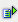The function ln (num) calculates the natural logarithm of a value.

Syntax: =ln(num)Example =ln(1) is 0 =ln(2.718282) is 1 =ln(22026,465795) is 10